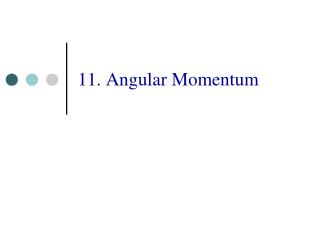Download Presentation11. Angular Momentum

Loading in 2 Seconds...

# 11. Angular Momentum - PowerPoint PPT Presentation

11. Angular Momentum. Angular Velocity and Acceleration Vectors. Rotation as a vector. A rotation has two attributes: An axis A magnitude Since the axis defines a direction , a rotation can be represented as a vector. Rotation – Angular Velocity.I am the owner, or an agent authorized to act on behalf of the owner, of the copyrighted work described.
Download Presentation## 11. Angular Momentum

An Image/Link below is provided (as is) to download presentation

Download Policy: Content on the Website is provided to you AS IS for your information and personal use and may not be sold / licensed / shared on other websites without getting consent from its author.While downloading, if for some reason you are not able to download a presentation, the publisher may have deleted the file from their server.

- - - - - - - - - - - - - - - - - - - - - - - - - - E N D - - - - - - - - - - - - - - - - - - - - - - - - - -
Presentation Transcript
1. 11. Angular Momentum

2. Angular Velocity and Acceleration Vectors

3. Rotation as a vector • A rotation has • two attributes: • An axis • A magnitude • Since the axis defines • a direction, a rotation can be • represented as a vector

4. Rotation – Angular Velocity We can use a convention, the right-hand rule, to define an angular velocity vector. Angular velocity is parallel to the rotation axis.

5. Rotation – Angular Acceleration The angular acceleration vector is in the direction in which the angular velocity is changing: Angular acceleration

6. The Cross Product The cross product of two vectors A and B produces a vector, of magnitude C= ABsinθ, that is perpendicular to the vectors A and B. The direction of the vector C is defined by the right-hand rule.

7. Torque and the Cross Product Torque can be represented as a vector using the cross product: Torque is perpendicular to both the position and force vectors.

8. Torque – Spinning Disk Consider a force applied at the edge of a disk. The resulting torque is given by

9. Angular Momentum Start with Newton’s 2nd Law Multiply by the position vector of the object

10. Angular Momentum Define angular momentum by But note that Why?

11. Angular Momentum & Torque Therefore, we can write Newton’s 2nd Law for rotational motion as

12. Angular Momentum – Example Angular momentum of a particle on spinning disk

13. Conservation of Angular Momentum

14. Conservation of Angular Momentum If the net external torque on a system of particles remains zero then

15. Example – Clutch (1)

16. Example – Clutch (2) Since the net external torque is zero: final angular momentum, Lf= initial angular momentum, Li

17. Example – Clutch (3) Equate final and initial: Lf= Li This expression is similar to that for a perfectly inelastic collision

18. Example – Clutch (4) Initial kinetic energy Final kinetic energy

19. Gyroscopes & Precession When the net torque is zero, the angular momentum is constant. Consequently, the axis of rotation of will remain fixed in space. This is the basis of a gyroscope.

20. Summary • Angular velocity, acceleration, torque and angular momentum can all be represented as vectors. • If the net torque is zero, the angular momentum is conserved. • Consequently, the axis of rotation remains fixed. Otherwise the axis will precess.